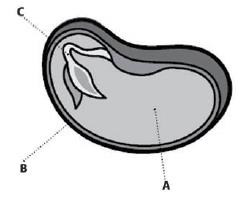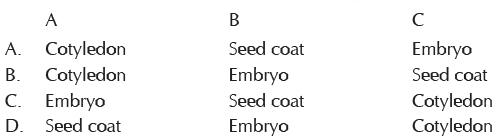Courses

# Olympiad Test : General Science - 2

## 20 Questions MCQ Test Science Olympiad for Class 5 | Olympiad Test : General Science - 2

Description
This mock test of Olympiad Test : General Science - 2 for Class 5 helps you for every Class 5 entrance exam. This contains 20 Multiple Choice Questions for Class 5 Olympiad Test : General Science - 2 (mcq) to study with solutions a complete question bank. The solved questions answers in this Olympiad Test : General Science - 2 quiz give you a good mix of easy questions and tough questions. Class 5 students definitely take this Olympiad Test : General Science - 2 exercise for a better result in the exam. You can find other Olympiad Test : General Science - 2 extra questions, long questions & short questions for Class 5 on EduRev as well by searching above.
QUESTION: 1

Solution:
QUESTION: 2

Solution:
QUESTION: 3

### Against which of the following vaccination is not available?

Solution:
QUESTION: 4

BCG vaccine is given to control:

Solution:
QUESTION: 5

Pneumonia is caused by:

Solution:
QUESTION: 6

As the temperature of a fixed amount of gas at constant volume decreases, its pressure .

Solution:
QUESTION: 7

As the volume of a fixed amount of gas at constant temperature decreases, its pressure .

Solution:
QUESTION: 8

Mist is often seen around ice cubes. Which of the following is a probable reason?

Solution:
QUESTION: 9

Which state of matter has particles able to slide past each other, yet still packed together?

Solution:
QUESTION: 10

The most common state of matter in the universe is called:

Solution:
QUESTION: 11

Choose the correct statement:

Solution:
QUESTION: 12

What is the definition of ENERGY?

Solution:
QUESTION: 13

Musical instruments and traffic on road are examples of:

Solution:
QUESTION: 14

The_____________a river possesses as a result of the movement of water.

Solution:
QUESTION: 15

This is based on mechanical energy.

Solution:
QUESTION: 16

This type of reproduction results in the organism producing an offspring genetically identical to it.

Solution:
QUESTION: 17

Seedless plants reproduce by forming:

Solution:
QUESTION: 18

This is the transfer of pollen grains to the female part of the plant.

Solution:
QUESTION: 19

Which is not a main part of a flower?

Solution:

The four main parts of a flower are the petals, sepals, stamen, and carpel (sometimes known as a pistil). If a flower has all four of these key parts, it is considered to be a complete flower.

So stem is not a main part

QUESTION: 20

Identify the parts labeled as A, B and C in the figure and select the correct option.Solution: